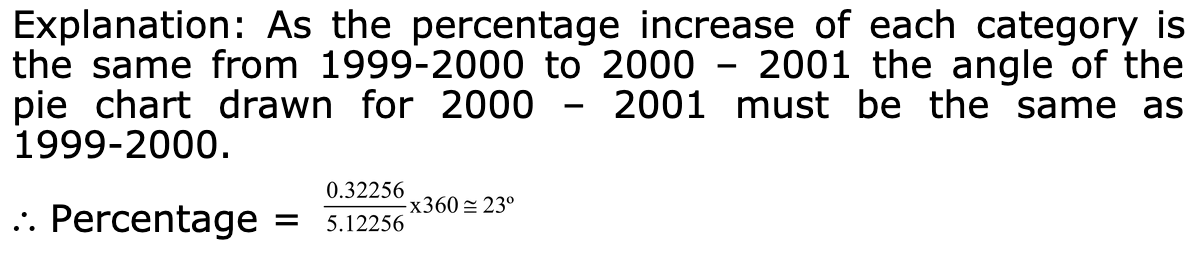CAT | ELITMUS Logical Questions

## ELITMUS Logical Questions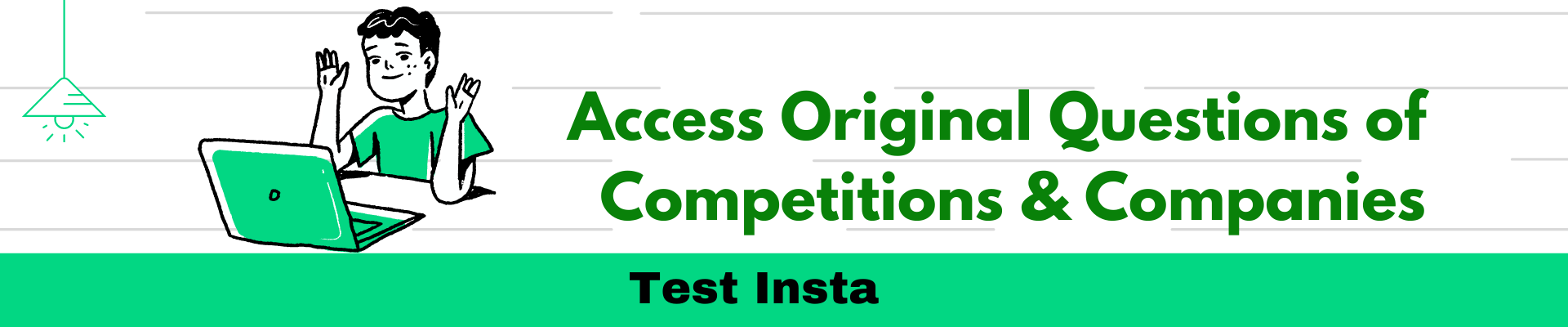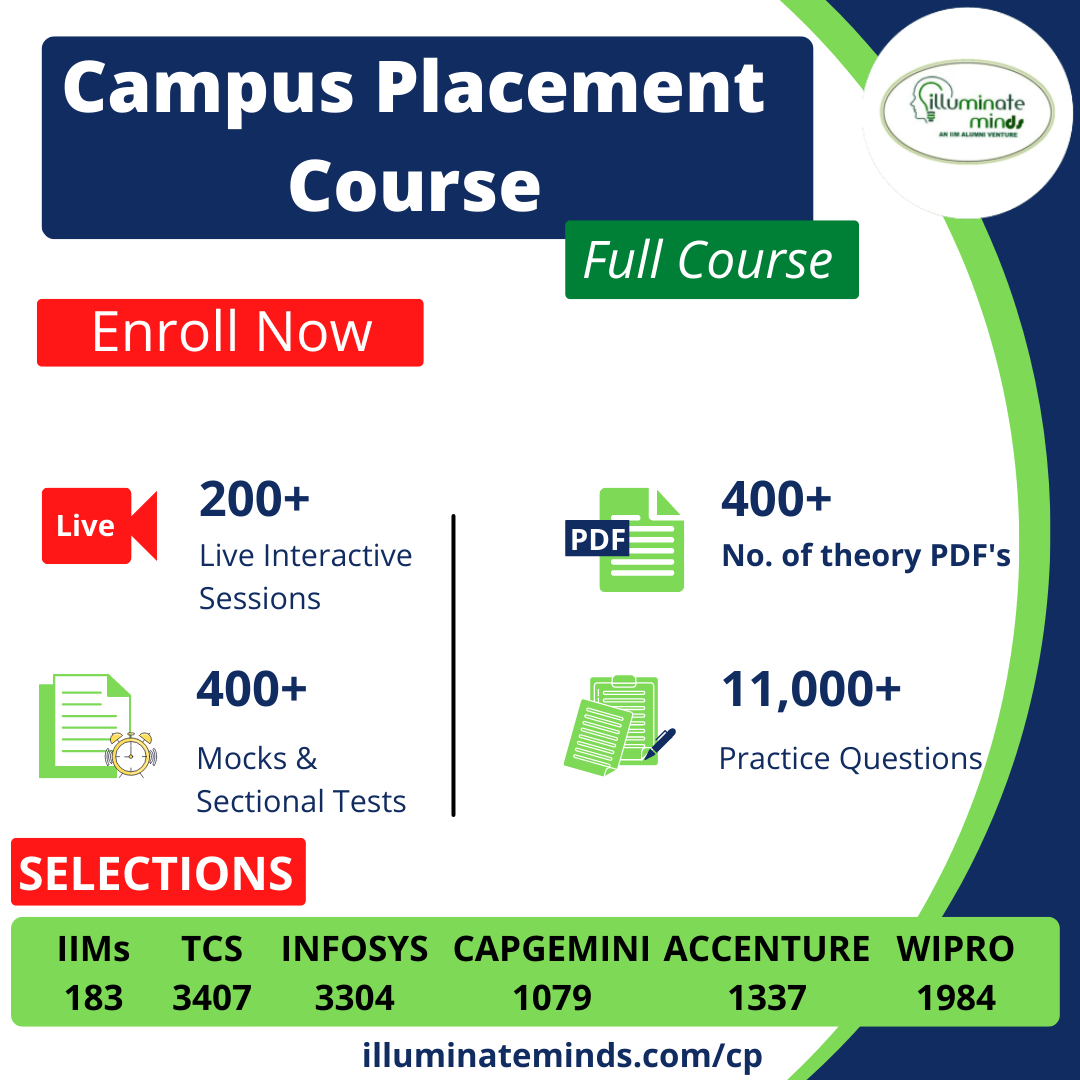Jobs By Batch
Jobs By Location
Jobs By Degree
Jobs By Branch
IT Jobs
Internships
Govt. Jobs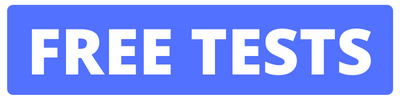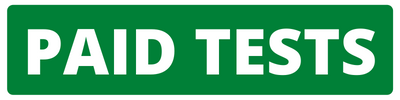• ##### Original Questions of IT Companies| All Topics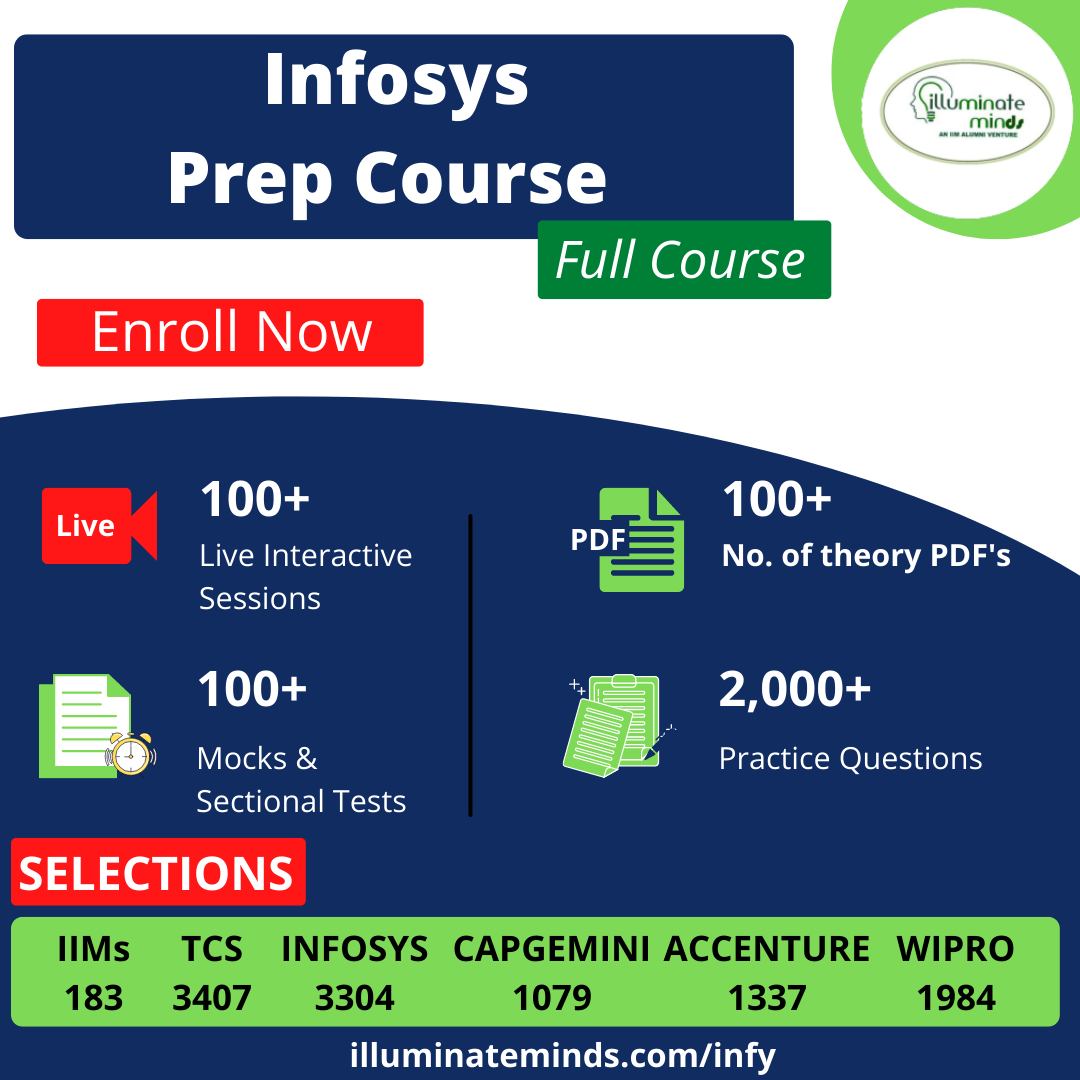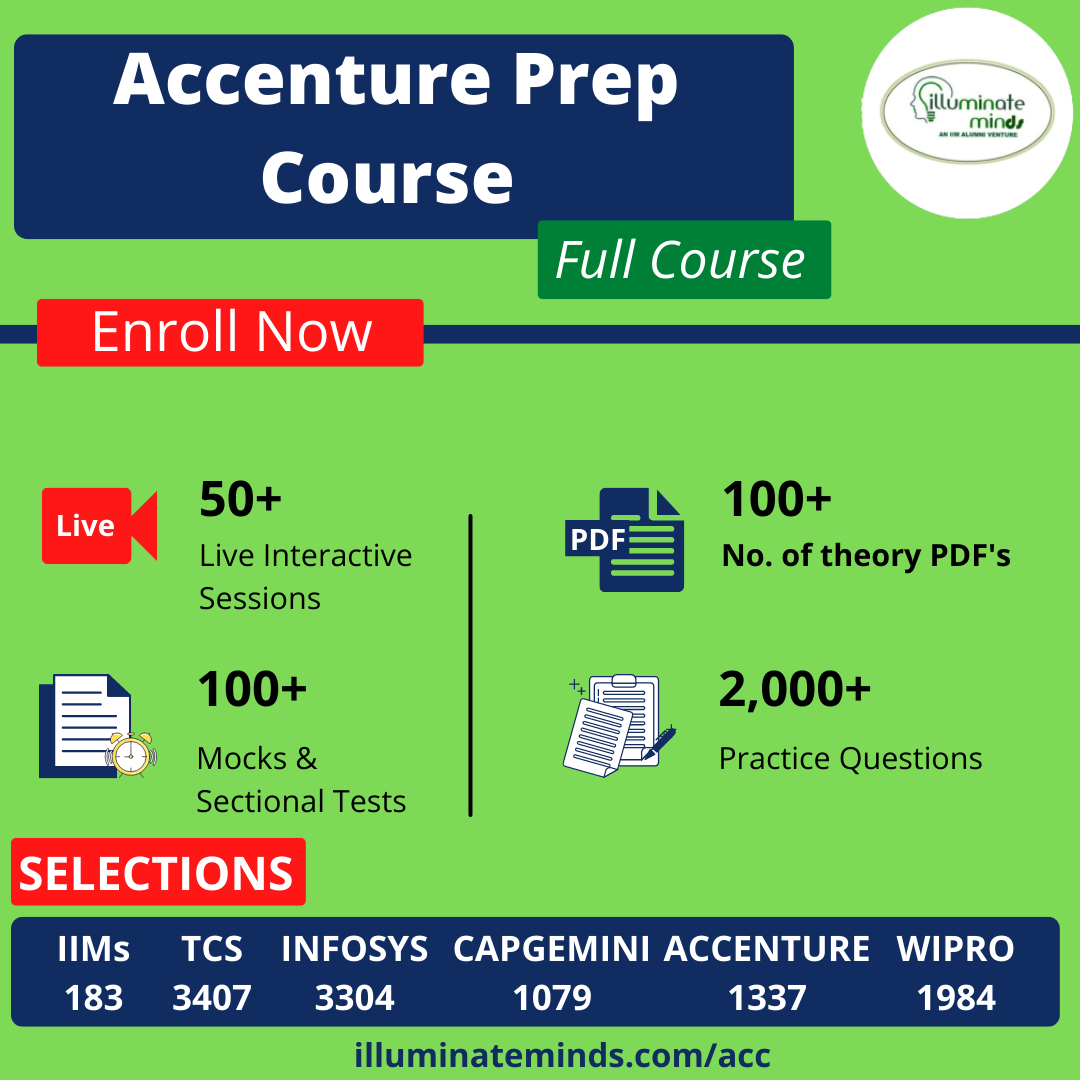### Quiz Begins Here

Q #1
:

#### Logical Question

Directions: These questions are based on the following pie chart and the table, which gives the details of expenses of an Excellent Management Training Institute in India (EMTI)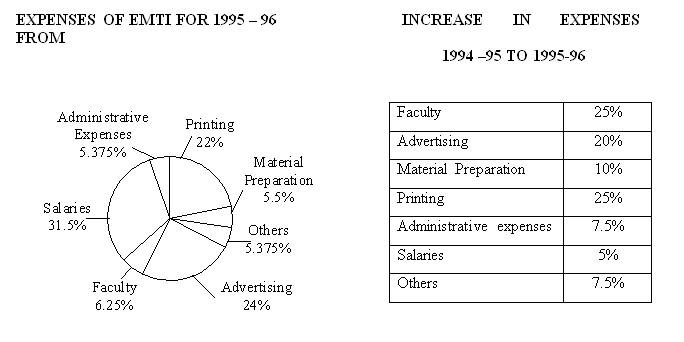If a pie-chart were to be drawn for the expenses in 1994-95 also, which expense would show the maximum drop in the angle from 1994-95 to 1995-96?
+

Explanation: The expense with the least increase shows the maximum drop in percentage share of the total.

Q #2
:

#### Logical Question

Directions: These questions are based on the following pie chart and the table, which gives the details of expenses of an Excellent Management Training Institute in India (EMTI)If the total expenses of EMTI in 1995-96 are Rs.16 lakhs, the total expenses in 1994-95 are closest to (in Rs. lacs).
+

Explanation: If the total expenses in 1995-96 is 100, the expenses 1994-95 is 87.6. Hence, if the expenses in 1995-96 is Rs. 16 lacs. The total expenses in 1994-95 = 16x0.876 = Rs.14 lacs.

Q #3
:

#### Logical Question

Directions: These questions are based on the pie charts given below.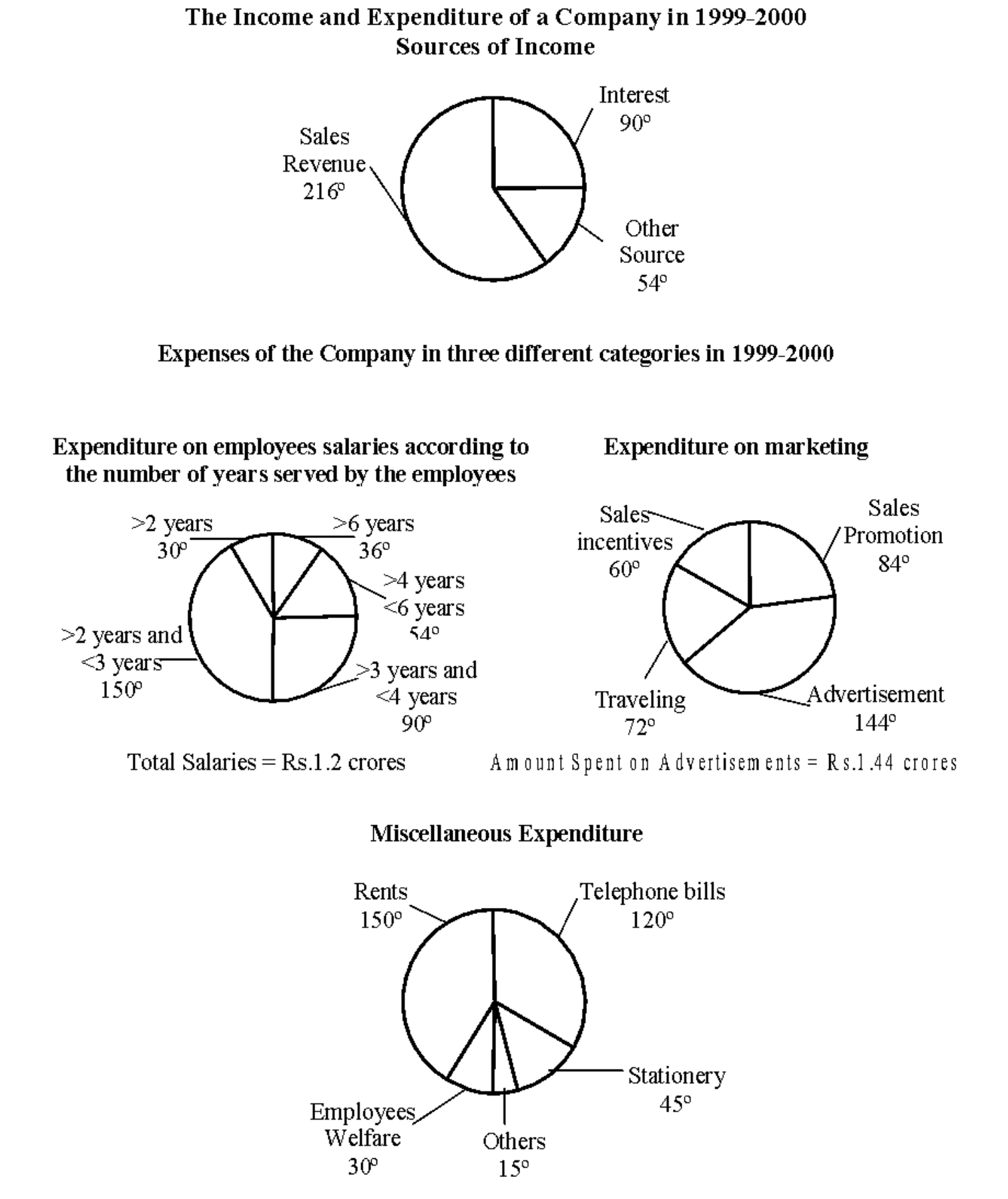The profitability of the company is defined as the profit expressed as a percentage of income and income is defined as the sum of the expenses and the profit. If the company made a profit of Rs. 1.0656 crores for the year, what is the profit percent of the company? (amount on Rent = 13.44 lakhs )
+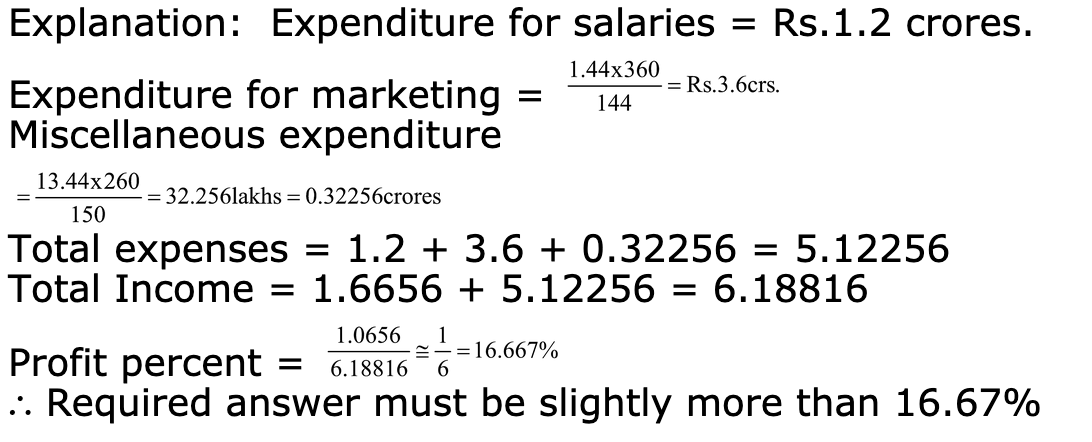Q #4
:

#### Logical Question

Directions: These questions are based on the pie charts given below.Using the data in question 6 and 7, the marketing expenses and the total salary of marketing employees put together form approximately what percentage of the total income of the company? (amount on Rent = 13.44 lakhs )
+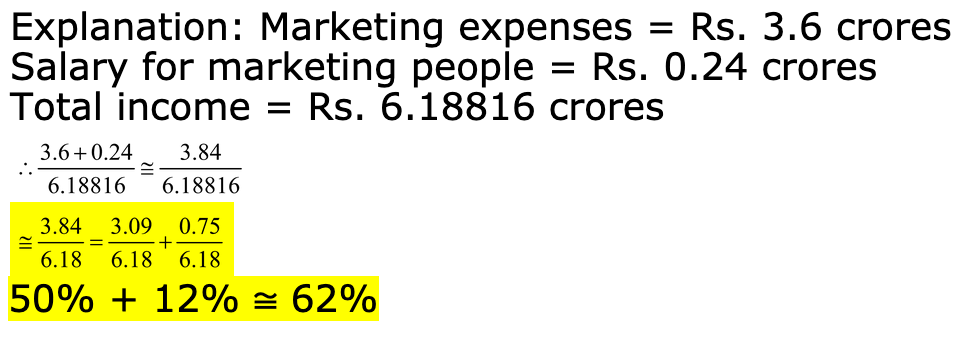Q #5
:

#### Logical Question

Directions: These questions are based on the pie charts given below.If a pie chart is drawn for the three categories of expenses in 2000-2001, considering the percentage increase in each category of expenditure the same as given in question 114, what is the approximate angle of miscellaneous expenditure?(amount on Rent = 13.44 lakhs )
+